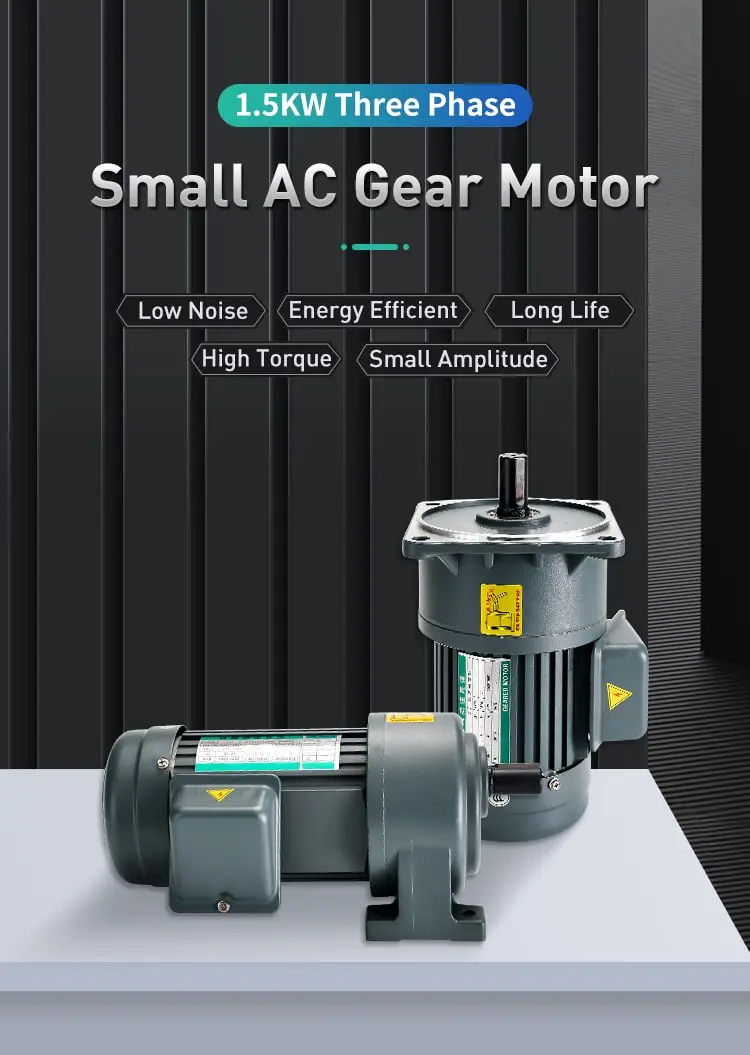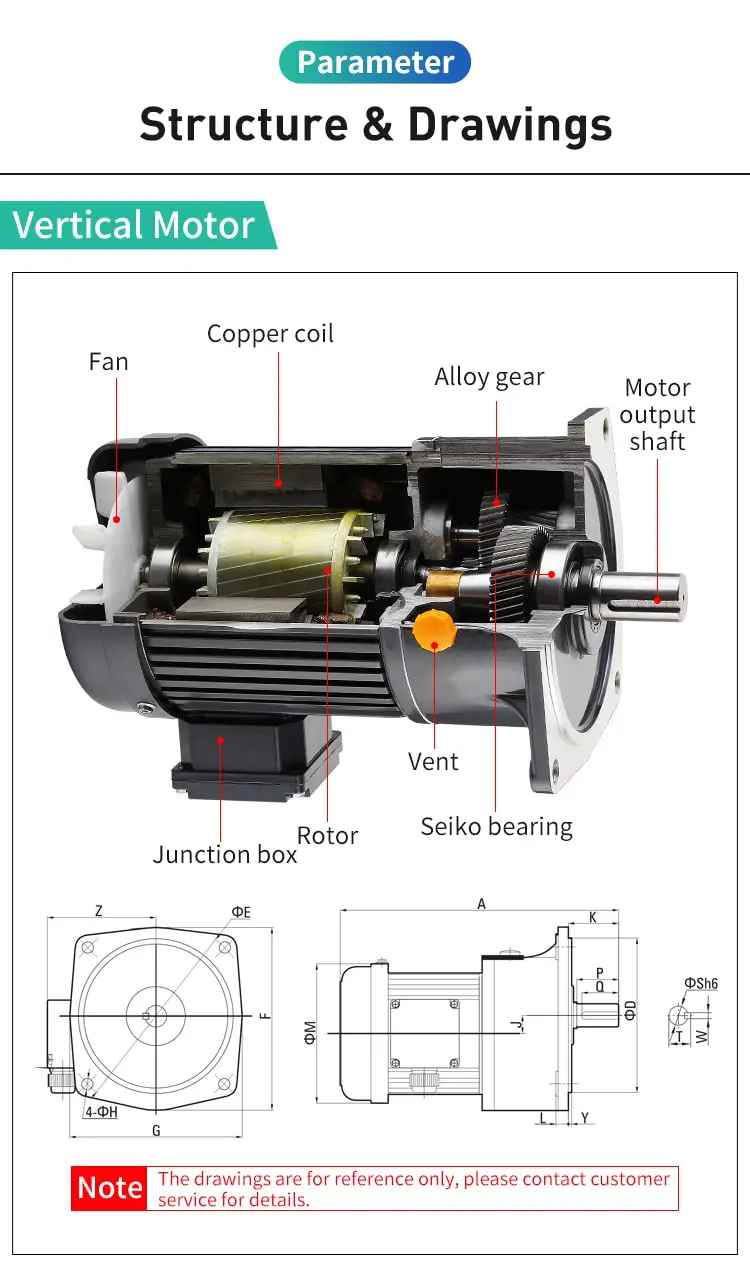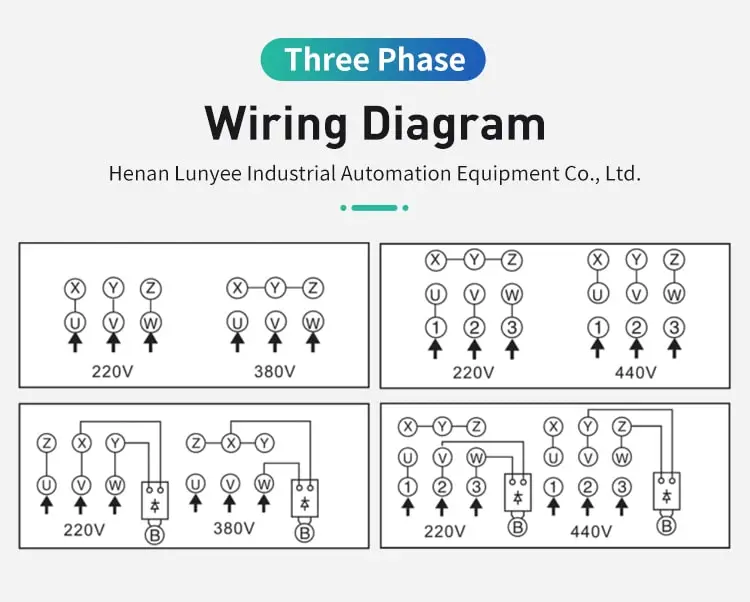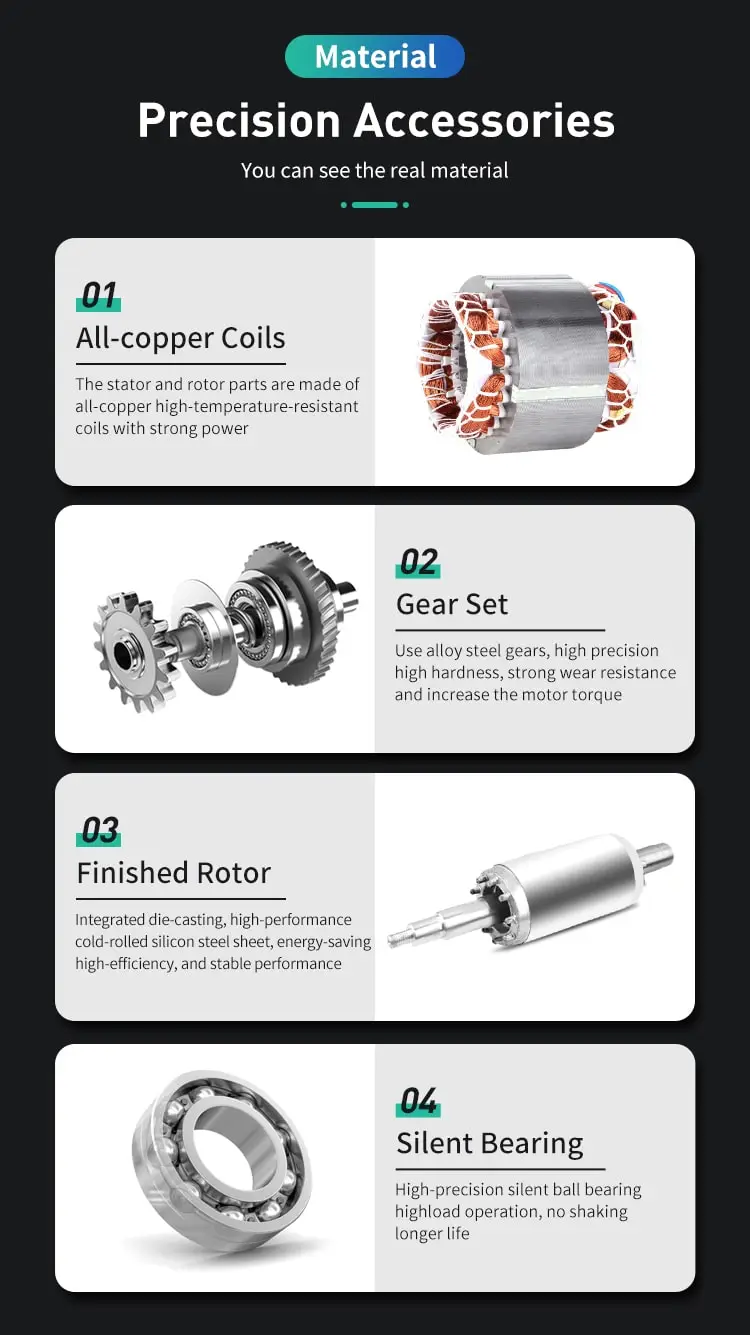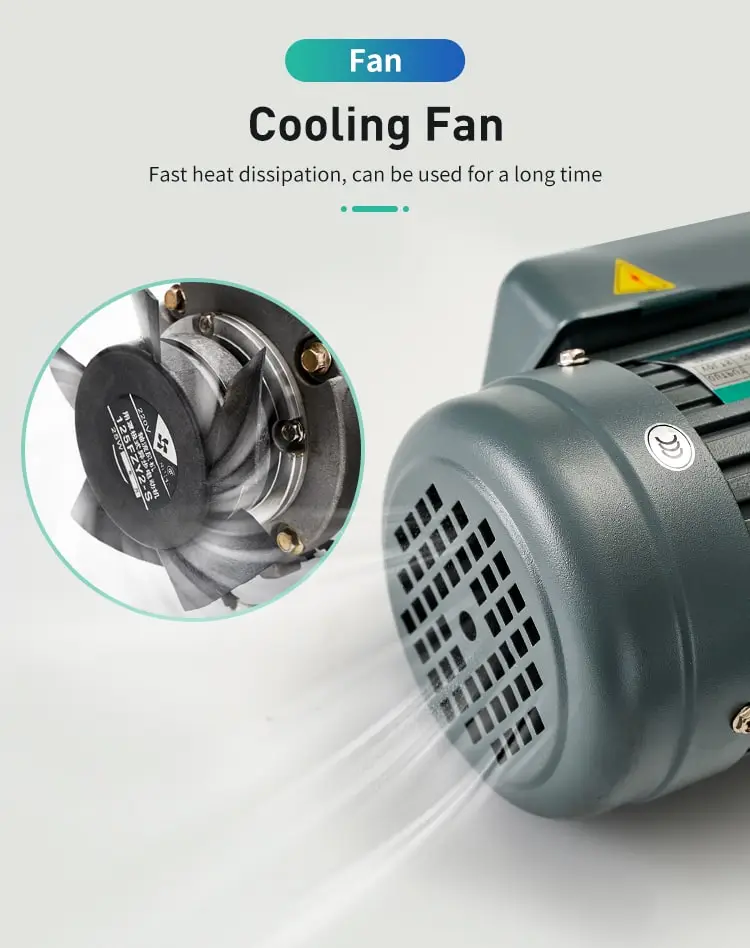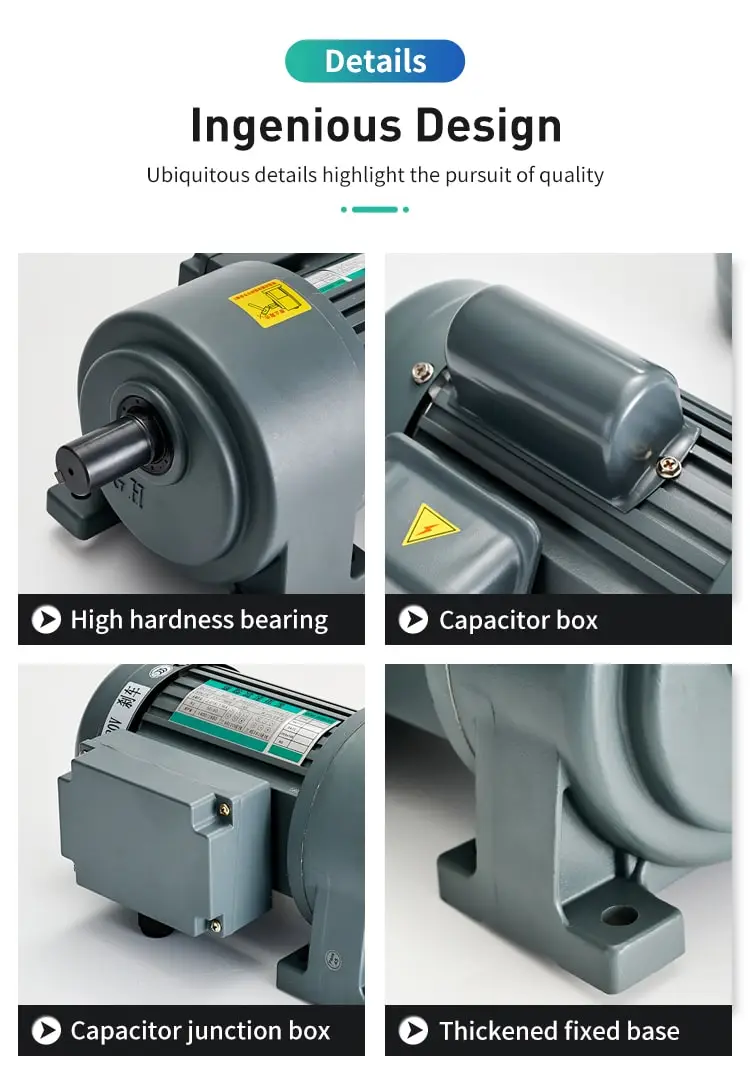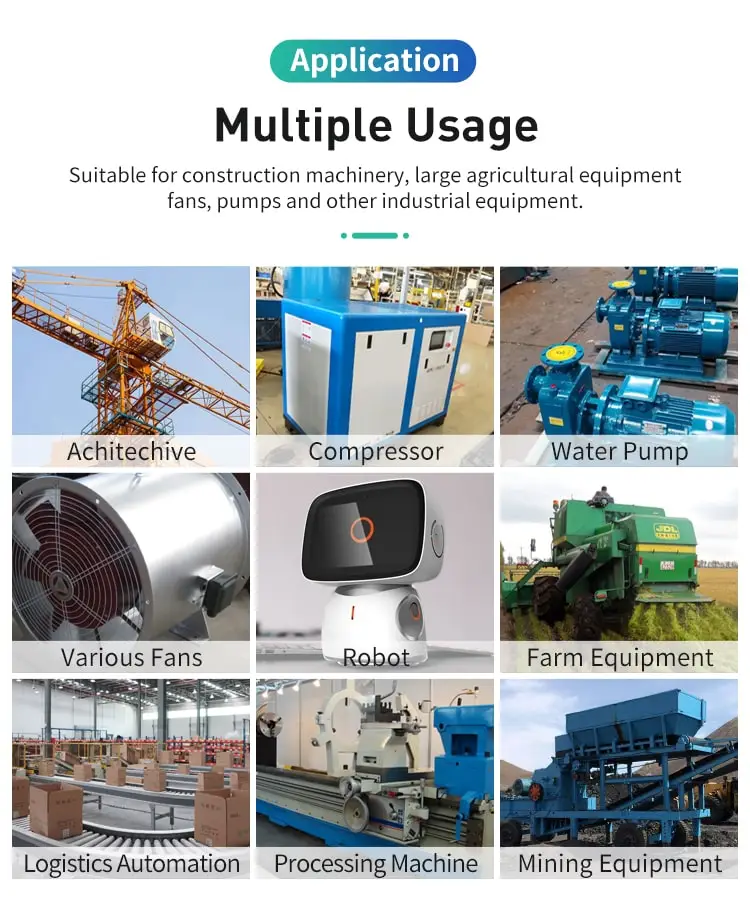By Jasmine 2022-12-22 17:49:50

#### Can an induction motor run at synchronous speed?

Whether an induction motor can run at synchronous speed depends on factors such as its rotor type and motor load, and in some cases, it can run synchronously.

An induction motor is a type of electric motor that uses electromagnetic induction to convert electrical energy into mechanical energy. In an induction motor, the rotor rotates because of the interaction between the magnetic fields from the stator and the rotor.

The rotational speed of an induction motor is not fixed, but rather it depends on the load on the motor and the frequency of the power supply. Typically, the rotational speed of an induction motor is slightly less than the synchronous speed, which is the speed at which the magnetic field from the stator rotates.

So, can an induction motor run at synchronous speed? The answer is yes and no. In some cases, an induction motor may be able to operate at synchronous speed, but in other cases it may not be possible.

One of the key factors that determines whether an induction motor can run at synchronous speed is the type of rotor it has. In a squirrel cage induction motor, for example, the rotor windings are made up of a series of conductive bars that are shorted at the ends. This creates a high resistance in the rotor, which makes it difficult for the rotor to reach synchronous speed.

On the other hand, in a wound rotor induction motor, the rotor windings are made up of a series of electromagnetic coils. This allows the rotor to have a lower resistance, which makes it easier for the rotor to reach synchronous speed.

In general, an Induction motor with a squirrel cage rotor is not able to run at synchronous speed, as the high resistance of the rotor windings makes it difficult for the rotor to reach the necessary speed. However, an induction motor with a wound rotor may be able to run at synchronous speed, depending on the load on the motor and the frequency of the power supply.

Another factor that determines whether an induction motor can run at synchronous speed is the load on the motor. In general, the higher the load on the motor, the slower the rotor will rotate. This means that if the load on the motor is too high, the rotor may not be able to reach synchronous speed, even if the rotor has a low resistance.

In summary, an induction motor can run at synchronous speed in some cases, but not in others. Whether an induction motor is able to run at synchronous speed depends on factors such as the type of rotor it has and the load on the motor. In general, an induction motor with a squirrel cage rotor is not able to run at synchronous speed, but an induction motor with a wound rotor may be able to do so